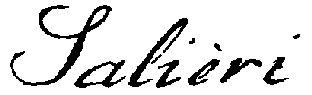[Contents] · [Home] · [Mail]

## SALIERI Language - Blocks

Blocks are sequences of SALIERI statements seperated by semicolons ";" and enclosed by single quotes `...´. (Note, that the closing single quotes "´" and "'" can be used synonymously.) Blocks are the most simple functional objects, they are used within control structures like loops and conditionals and for function and macro bodies.

A block is really a special function which has generally no parameters and no local workspace and it is always evaluated within the context where it was declared. Thus, object names appearing in a block are always treated as if they were found just outside the declaration of the block (see example below).

There are two different types of blocks: statement blocks and expression blocks. Statement blocks contain only statements; when evaluated, these statements are executed, but there will be no result value. Examples for statement blocks are loop bodies and the bodies of procedural functions (i.e., functions which don't return a value). Expression blocks, on the other hand, end with an expression or a return statement, which, when evaluated, determines the result value of the block. Examples for these are loop conditions such as `i<10' or the bodies of functions which return a value.

Blocks are treated as ordinary objects of type "function", as such they can be created, copied, deleted, and passed as function parameters. Block objects are executed using the predefined function apply (see example below).

A special case of a statement block is `', the empty block. When executed, it has no effect (and of course returns no value).

Attention!

• Make sure that evaluated statement blocks are never used where a value is expected (as on the right hand side of assignments or in parameter lists of function calls). Keep in mind that evaluating a statement block is equivalent to executing a number of statements.
• Take care that evaluated expression blocks only occur where a value is actually expected. Keep in mind that when evaluated, expression blocks are equivalent to the resulting object value.
• Don't try to pass additional parameters when executing blocks using apply. This will result in an error condition ("Unassigned element on stack").
• Note the difference between an expression like 1+2*3 and an expression block `1+2*3'. Whereas the former is equivalent to the integer value 7, the latter is a block object, which evaluates to 7 when executed.

Example:
> b := `x:=3' (* a simple statement block *)
> type(b)
"function"
> b
Result= `x:=3'
> x := 1;
> x
Result= 1
> apply(b) (* execute the block *)
> x
Result= 3
> be := `x>=3' (* a simple expression block *)
> be
Result= `x>=3'
> apply(be)
Result= TRUE
> apply(`x') (* evaluating another expression block *)
Result= 3
> if(be, `x:=0', b);
> x
Result= 0
> f := FUNC(2, `if(`apply(\$1)',`apply(\$2)')');
> f(`x<1', `x:=1; write("x=",x)')
x=1
> x (* x in `x:=1' refers to global workspace! *)
Result= 1
> f2 := FUNC(1, `if(`apply(\$1)',`x:=2; write("x=", x)')');
> f2(`x=1')
x=2
> x (* x in `x:=1' refers to local workspace of f2! *)
Result= 1

See also: FUNC, MACRO, apply, return, function (Object Type).

[Contents] · [Home] · [Mail]
© sic!systems, page frame designed by hh; this page has been automatically generated from the SALIERI Documentation Database.